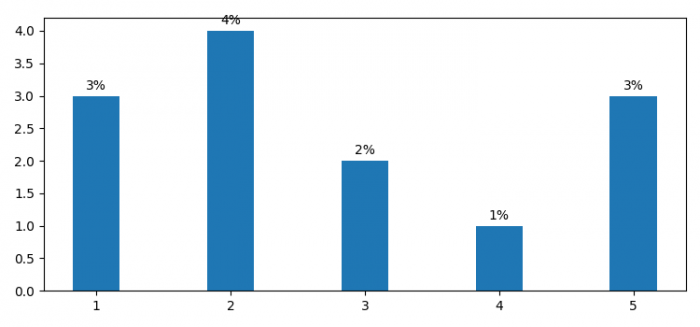# How to display percentage above a bar chart in Matplotlib?

To display percentage above a bar chart in Matplotlib, we can take the following steps −

• Set the figure size and adjust the padding between and around the subplots.
• Create x and y data points; initialize a variable, width.
• Create a figure and a set of subplots using subplots() method.
• Add bars with x and y data points.
• Iterate bars patches; put text over the bars using text() method.
• To display the figure, use show() method.

## Example

from matplotlib import pyplot as plt
import numpy as np

plt.rcParams["figure.figsize"] = [7.50, 3.50]
plt.rcParams["figure.autolayout"] = True

x = [1, 2, 3, 4, 5]
y = [3, 4, 2, 1, 3]

width = 0.35
fig, ax = plt.subplots()

pps = ax.bar(x, y, width, align='center')

for p in pps:
height = p.get_height()
ax.text(x=p.get_x() + p.get_width() / 2, y=height+.10,
s="{}%".format(height),
ha='center')

plt.show()

## Output